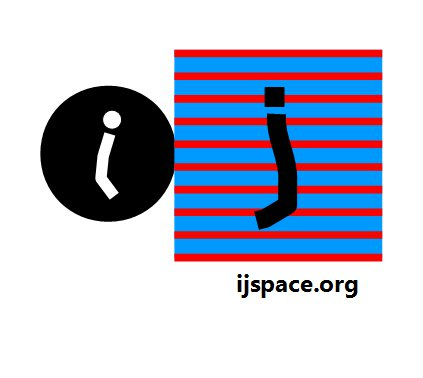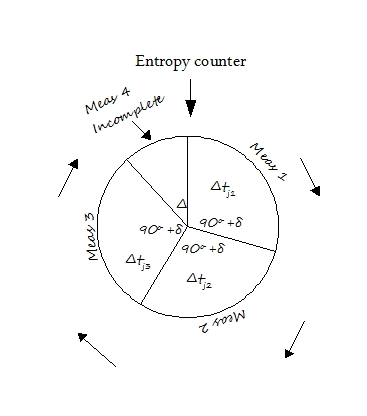Home Summary: We discuss the structure formation in discrete measurement space as measured by the macroscopic observer ObsM.  We develop the description for the base-states and "sense" property and their combinations into structures.While describing dimensions we had three PE1j measurements and one partial measurement before the entropy counter was reset.  The PE1j measurements represented the portion of  information, which formed a stable structure, whereas the partial measurement represented the information with infinitesimal life-time in measuring observer's time-frame.  For a macroscopic observers the structures formed consist of PE1j measurements and their combinations.       We also introduce the what we may call "sense" property.  We clock the initial state when the measurements begun for the observer in j-space as state.  The further states are formed from the initial state in j-space.  We will have two possibilities: (i) we have the motion towards state from state represented as <↓>, (ii) we have the motion away from state towards state represented as <↑> .       We can describe a measurement in terms of PE1j measurements and "sense" states which combine together form the "base" states. There are three PE1j measurement states possible with EM tool before the entropy change, each with two “sense” states.  Therefore we have six possibilities represented as <↕>1, <↕>2 , <↕>3.        We can think of eight possible measurement states in discrete j-space using EM tool, upon which a macroscopic observer can build his observations by making various assumptions.  These states are,   <↓↓↓>,  <↑↓↓>, <↓↑↓>, <↓↓↑>, <↑↑↑>, <↓↑↑>, <↑↓↑>, <↑↑↓>.                                (i) Step-1 Each arrow represents a PE1j measurement with one of two possible sense state.  For example let us restrict the measurement of  Meas-1 in the direction from to or <↑> only, due to entropy.  In that case the states represented by (i) are measured as, <↓↓↓>  = <↑↓↓>, <↑↓↓> unchanged, <↓↑↓> = <↑↑↓>, <↓↓↑> = <↑↓↑>, and <↑↑↑> unchanged, <↓↑↑> = <↑↑↑>, <↑↓↑> unchanged, <↑↑↓> unchanged.         (iia) Thus a degeneracy is introduced due to measurement limitations and four states result out of eight base states.  The bar under arrow <↑> represents a restriction or a symmetry due to observer's limitations.  We can place similar restriction on Meas-2 and Meas-3 and obtain the following, <↓↓↓> = <↓↑↓>, <↓↑↓> unchanged, <↓↓↑> = <↓↑↑>, <↑↓↓> = <↑↑↓>, <↑↑↑> unchanged, <↓↑↑> unchanged, <↑↓↑> = <↑↑↑>, <↑↑↓> unchanged,   (iib) and <↓↓↓> = <↓↓↑>, <↓↑↓> = <↓↑↑>, <↓↓↑> unchanged, <↑↓↓> = <↑↓↑>, <↑↑↑> unchanged, <↓↑↑> unchanged, <↑↓↑> unchanged, <↑↑↓> = <↑↑↑> .     (iic) An important point to note is that Meas-1, Meas-2, and Meas-3 are not identical. Step-2 We can place similar constraints on two measurements simultaneously.  We get, <↓↓↓> = <↑↑↓>, <↓↑↓> = <↑↑↓>, <↓↓↑> = <↑↑↑>, <↑↓↓> = <↑↑↓>, <↑↑↑> unchanged, <↓↑↑> = <↑↑↑>, <↑↓↑> = <↑↑↑>, <↑↑↓> unchanged,            (iiia) and <↓↓↓>  =  <↓↑↑>,  <↓↑↓>  =  <↓↑↑>, <↓↓↑>  =  <↓↑↑>, <↑↓↓>  =  <↑↑↑>, <↑↑↑>  unchanged, <↓↑↑>  unchanged, <↑↓↑>  =  <↑↑↑>,  <↑↑↓>  =  <↑↑↑>,       (iiib) and <↓↓↓>  =  <↑↓↑>,  <↓↑↓>  =  <↑↑↑>,  <↓↓↑>  =  <↑↓↑>,  <↑↓↓>  =  <↑↓↑>,       <↑↑↑>  unchanged, <↓↑↑>  =  <↑↑↑>,  <↑↓↑>  unchanged, <↑↑↓>  =  <↑↑↑>.  (iiic) Step-3 Similarly if we place entropy constraint on all three states then all eight states are measured as, <↓↓↓> = <↑↑↑>,  <↓↑↓> = <↑↑↑>, <↓↓↑> = <↑↑↑>, <↑↓↓> = <↑↑↑>,       <↑↑↑> unchanged, <↓↑↑> = <↑↑↑>, <↑↓↑> = <↑↑↑>, <↑↑↓> = <↑↑↑>.       (iv)       Let us consider <↑↑↑> first.  The state <↑↑↑> is a PE1j state in which all arrows point towards state i.e. it is a low energy state.  We have described a measurement structure with 2, 6, 12 and finally 8 level measurement degeneracy for <↑↑↑> state, which represents a state which has tendency to move away from δi or state.  Therefore an observer using EM as measurement signal is likely to measure PE1j structures in the sequence shown as the measurements move away from δi.       The other states appearing in above configuration have at least one <↓> state and hence EM signal which represents a low energy configuration (incomplete PE1j measurement), will not detect these states unless additional energy is provided.  These states will be identified only after comparison with experimental results.             We note that we have been describing the structure (PE1j)  and signal (PE < 1j) as components of the same information space, hence they are interlinked.  For different q values the description of PE1j structures and the signal characterizing the interaction between these structures will change.        We have shown that there are eight possible basic states based on “Sense” and EM measurements.  They are repeated below: <↓↓↓>,  <↑↓↓>, <↓↑↓>, <↓↓↑>, <↑↑↑>, <↓↑↑>, <↑↓↑>, <↑↑↓>, and with entropy considerations there are twenty seven possible measurement states as described below, <↓↓↓>, <↑↓↓>, <↓↑↓>, <↓↓↑>,     <↑↑↑>, <↓↑↑>, <↑↓↑>, <↑↑↓>, (Eight States),   <↑↓↓>, <↑↑↑>, <↑↑↑>, <↑↓↑>, <↓↑↑>, <↓↑↑>, <↑↑↓>, <↑↑↓>, <↑↓↑>, <↑↑↑>, <↓↑↓>, <↓↓↑>, (Twelve States),   <↑↑↑>, <↑↑↑>, <↑↑↑>, <↑↑↓>, <↓↑↑>, <↑↓↑>, (Six states),   <↑↑↑>, (One state).   The observer ObsM based on its capability will measure a macroscopic structure based on a combination of these states in j-space.  If a composite structure has a property, "charge" for example, then the base state is likely to have 1/3rd of the property measured.       It is very tempting to jump to a conclusion that the structure being described correspond to quarks, s, p, d, f orbits or that <↑↑↑> represents an electron.  We must exercise caution as we have not really matched energy values or known experimental data with the description in the measurement space.  All we can say at this point is that the structures similar to those developed in abstract, are likely to be measured by a macroscopic observer in (t, x, y, z) space.  We will discuss the interaction in j-space next."So long as big and small are merely relative concepts, it is no help to explain the big in terms of small." - Dirac, The Principles of Quantum Mechanics, Oxford University Press. "..The quantum field theory of weak, electromagnetic and strong interactions, is nothing more than a low energy approximation to a much deeper and quite different underlying field theory." - Steve Weinberg, Dirac's Memorial Lectures 1986.